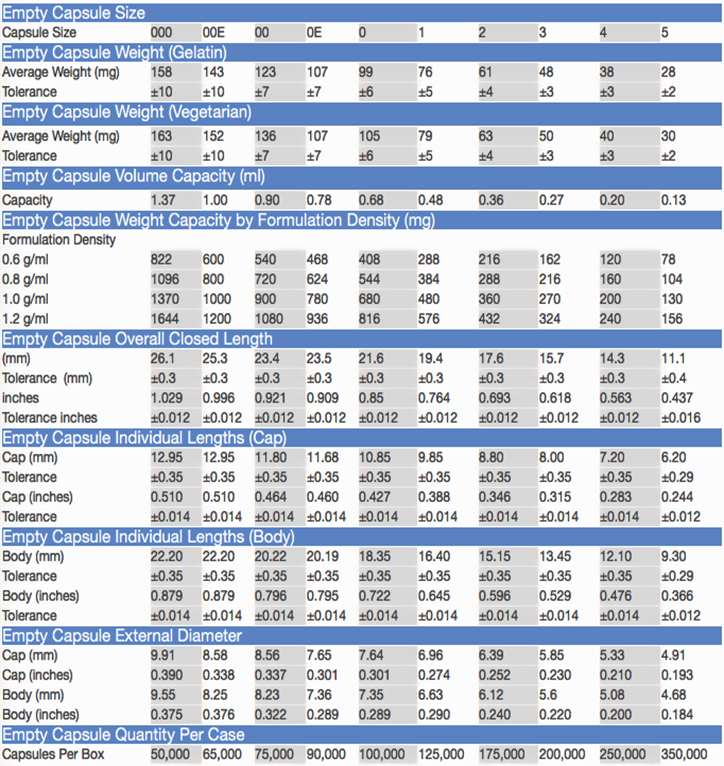# Which Capsule is Right For You: A Guide to Choosing the Right Capsule Size for Your Needs

## Understanding The Capsule Sizing System

One of the most common questions our customers ask is about capsule sizing. The capsule sizing system is a little complicated, and confusing at times, so we’ve written this guide to help you understand the ins-and-outs of capsule sizing.

See our chart below, we’ll refer back to it throughout this piece.

### Capsule Numeric Sizing System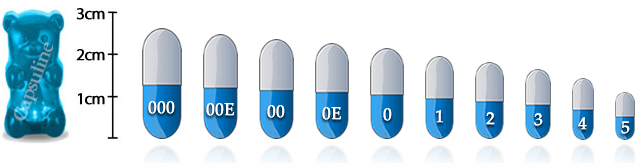Capsules are categorized by sizes ranging from 5 (smallest) to 000 (largest.)  The numeric designation used for capsule sizes is inversely correlated to the size of the capsule. These sizes are standardized regardless of the capsule material (gelatin or vegetable derived).

The larger the number, the smaller the capsule. Using this standard sizing system allows us to ensure that both encapsulators and consumers receive the proper capsule for their needs.

## What Size Capsule Do I Need?

Some of our most frequently asked questions revolve around finding the right capsule size. Often, customers will ask us something along the lines of, “How many capsules would it take to hold one teaspoon of contents?” or “What capsule size do I need?” Sometimes the answer to these types of questions can get a bit complicated. For example, let’s take a look at the question of encapsulating a teaspoon of material.

One teaspoon fills about 7 “0” capsules and about 5 “00” capsules. The weight of the material you’re filling the capsules with will depend on its density.  Density varies widely due to the properties of the material you’re filling the capsules with, eg. powders, liquids, granules, herbs, etc. This density must be calculated in order to know how much material will fit in a certain sized capsule. Which is why there is no single answer to the question of, “Which capsule size is right?”## So, how much space do you need?

In order to choose the right capsule size for your application, you need to know two things, the formulation density and the mass (total weight) of the product you want to encapsulate.

In making these determinations, you may use either US Customary or Metric units of measurement. You can find some of the most common conversions between the two units of measurement in the chart to the right.

Regardless of which unit of measurement you choose, make sure to use the same system of measurement throughout any calculations.  Here we will use the Metric System.

In order to determine a formulation’s density, we use the following formula: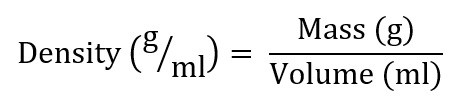Formulation Density Volume – Find the density of your product by dividing its mass by its volume; see formula below.

Mass (total weight) – Find the weight of your product by simply weighing it on an accurate scale.

Volume– The amount of the substance that is enclosed within the capsule. This is found my measuring with a measuring cup or spoon.

Grams and Milliliters– Grams are a measure of mass while ml is a measure of volume. Thus, we describe formulation density, in consideration of capsule capacity, in g/ml to indicate the density is derived from dividing the mass (g) by the volume (ml.)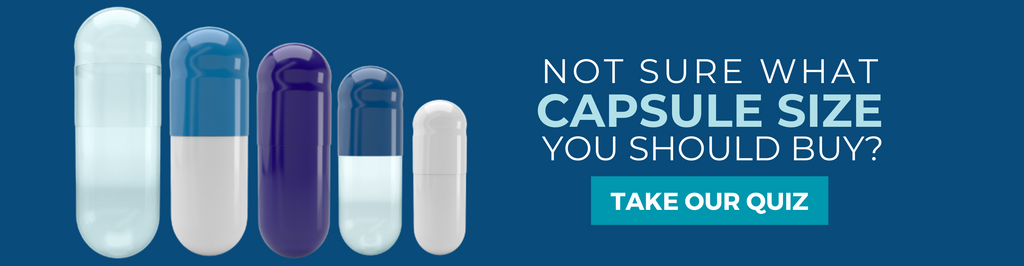## Find Formulation Density By Mass and Volume

### What you’ll need: a scale and a measuring cup or spoon

Find Formulation Density:

1. Measuring mass: Fill a container with your product, weigh it, and record the number. Remove your product from the container and weigh the container. Subtract the weight of the container from the total weight.
2. Measuring volume: Volume is the space the product fills, so use a measuring cup or spoon to determine your products’ volume.
3. Divide the mass by the volume to arrive at your formulation density. This will give you a result in units like this:  1 g/ml (grams per milliliter)

### A Real-World Example

Say you need to encapsulate 600mg (.6g) of turmeric powder and need to know what capsule to buy.

1. Determine mass – Milligrams are a measure of mass, so you have the first part of the equation to start with.
2. Determine volume – Place .6 grams of turmeric powder into a measuring spoon and determine how much space it fills by the demarcations on the spoon. For demonstration purposes, we’ll say that the volume is 1ml
3. Divide .6 gram by the 1ml to give you the grams per milliliter (g/ml)

Mass:                 .6 g

——————       = .6 g/ml

Volume:      1 ml (1/2 tsp)

Thus: Formulation density = .6 g/ml

Next, using our chart, locate the density that is closest to .6 g/ml. Then locate the capsule with the capacity closest to your total weight, in this case 600mg. In this case, a size 00E (or larger) capsule will accommodate your needs.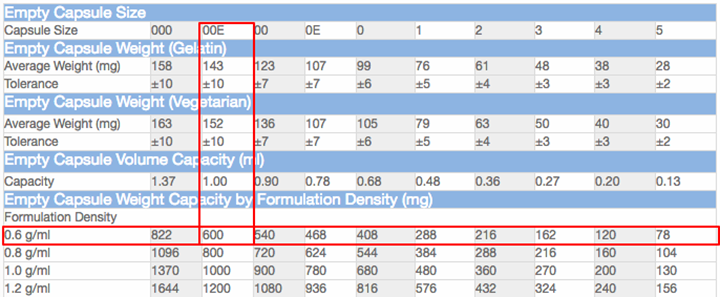## Sizing Chart

### A few notes on reading our sizing chart.

The capsule cap is the shorter half, or top, of the capsule. The capsule body is the longer half, or bottom, of the capsule.  And cases of capsules are wholesale quantities that aren’t separated into smaller packaging.

Capacity (ml) means the capacity of the capsule in units of volume. While External Diameter refers to the width of the capsule at its widest point (the center.)

Weight Capacity by Formulation Density (mg) is the capacity of a capsule in units of mass, taking into consideration how the density of a formula can affect how much of it can be contained in the empty capsule.